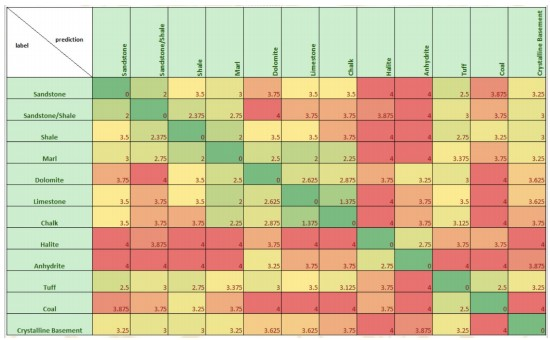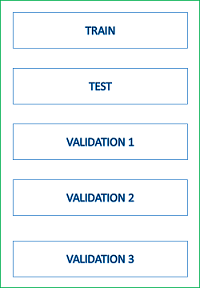# Modelling.ipynb

Olawale

This notebook covers the modelling techniques used for getting optimal prediction results.

## Data Cleaning and Preparation¶

This involves the necessary steps and processing to get both the train and test datasets in an acceptable form for the machine learnning algorithm:

• Creating validation data sets
• Selecting interested logs
• Resolving missing values
• Encoding categorical variables
• Data augmentation
from google.colab import drive
drive.mount('/content/drive', force_remount=True)
cd /content/drive/MyDrive/TRANSFORM-2021
import numpy as np
import random
import pandas as pd
import xgboost as xgb
from sklearn.model_selection import StratifiedKFold
from sklearn.metrics import accuracy_score, f1_score
traindata = pd.read_csv('./data/train.csv', sep=';')
testdata = pd.read_csv('./data/leaderboard_test_features.csv.txt', sep=';')
A = np.load('./data/penalty_matrix.npy')   # panlty matrix used for scoring
A

Scoring matrix for petrophysical interpretationdef score(y_true, y_pred):

'''
custom metric used for evaluation
args:
y_true: actual prediction
'''

S = 0.0
y_true = y_true.astype(int)
y_pred = y_pred.astype(int)
for i in range(0, y_true.shape):
S -= A[y_true[i], y_pred[i]]
return S/y_true.shape

### Creating Validation Sets¶

Validation sets are created to properly evaluate changes made on the machine learning model. This is important to prevent overfitting the open test data since the blind test is used as the final determiner. So it is important to build ML models that will generalise better on unseen wells. Having no idea how the blind wells would come (geospatial distribution, logs presence), there was no specific guide in selecting train wells, so wells were randomly selected from the train to create two validation sets (each comprising of 10 wells).

train_wells = traindata.WELL.unique()
print(f'Initial total number of train wells: {len(train_wells)}')
np.random.seed(40)
valid1 = random.sample(list(train_wells), 10)   #randomly sample 10 well names from train data

QC to remove valid1 wells from train wells to prevent having same well(s) in the second validation data

train_wells = [well for well in train_wells if not well in valid1]
print(f'Number of wells left: {len(train_wells)}')
valid2 = random.sample(list(train_wells), 10)

train_wells = [well for well in train_wells if not well in valid2]
print(f'Number of wells left: {len(train_wells)}')
print(len(valid1), len(valid2))
validation_wells = set(valid1 + valid2)
print(len(validation_wells))

Let's proceed to getting the validation data from the train data set and dropping them to prevent any form of data leakage.

def create_validation_set(train, wells):

'''
Function to validation sets from the full train data using well names
'''

validation = pd.DataFrame(columns=list(train.columns))

for well in wells:
welldata = train.loc[train.WELL == well]
validation = pd.concat((welldata, validation))

return validation
# using function to get data for validation wells

validation1 = create_validation_set(traindata, valid1)
validation2 = create_validation_set(traindata, valid2)
# total validation data

validation = pd.concat((validation1, validation2))
validation.shape, validation1.shape, validation2.shape
# dropping validation data from train data

new_train = pd.concat([traindata, validation, validation]).drop_duplicates(keep=False)
print(f'Previous train data shape: {traindata.shape}')
print(f'New train data shape: {new_train.shape}')
# QC to ensure there are no data leakage

previous_rows = traindata.shape
new_train_rows = new_train.shape
validation_rows = validation.shape

print(f'Number of previous train data rows: {previous_rows}')
print(f'Validation + validation rows: {validation_rows+new_train_rows}')
# to confirm we still have all samples of the labels in the train data set

new_train.FORCE_2020_LITHOFACIES_LITHOLOGY.value_counts()

Now we can proceed to other preparation procedures.

Logs were selected based on user's desire to use them for training. The confidence logs was dropped as this was absent in the test logs as the ML models need the same set of wells used for training in making predictions on the test wells. Other absent logs and logs with low percentage of values from the combined test data are also dropped. This has previously been visualized in the EDA notebook. A cut off of 30% may be selected as criteria for dropping the logs. Let's take a look at that!

print(f'Percentage of values in test logs:')
100 - testdata.isna().sum()/testdata.shape*100

For better and faster processing, the train, validation and test data sets will be concatenated and processed together as we need these data sets to be in the same formats to get good predictions out of the ML model. But let's have it in mind that the RSHA, SGR, DCAL, MUDWEIGHT, RMIC and RXO will be dropped from the wells. Let's proceed.

Let's extract the data sets indices that will be used for splitting the features and targets into their respective datasets after prepration is complete. We will also be extracting the train target

ntrain = new_train.shape
ntest = testdata.shape
nvalid1 = validation1.shape
nvalid2 = validation2.shape
nvalid3 = validation.shape

df = pd.concat((new_train, testdata, validation1, validation2, validation)).reset_index(drop=True)

So the combined dataframe for preparationdf.shape

The procedure below is used to extract data needed for the augmentation procedure to be performed after every other preparation has been done

#making a copy of the dataframes

train = new_train.copy()
test = testdata.copy()
valid1 = validation1.copy()
valid2 = validation2.copy()
valid = validation.copy()
# extracting the data sets well names and depth values needed for augmentation

train_well = train.WELL.values
train_depth = train.DEPTH_MD.values

test_well = test.WELL.values
test_depth = test.DEPTH_MD.values

valid1_well = valid1.WELL.values
valid1_depth = valid1.DEPTH_MD.values

valid2_well = valid2.WELL.values
valid2_depth = valid2.DEPTH_MD.values

valid_well = valid.WELL.values
valid_depth = valid.DEPTH_MD.values

Now let's extract the data sets labels and prepare them for training and validation performance check.

lithology = train['FORCE_2020_LITHOFACIES_LITHOLOGY']
valid1_lithology = valid1['FORCE_2020_LITHOFACIES_LITHOLOGY']
valid2_lithology = valid2['FORCE_2020_LITHOFACIES_LITHOLOGY']
valid_lithology = valid['FORCE_2020_LITHOFACIES_LITHOLOGY']

lithology_numbers = {30000: 0,
65030: 1,
65000: 2,
80000: 3,
74000: 4,
70000: 5,
70032: 6,
88000: 7,
86000: 8,
99000: 9,
90000: 10,
93000: 11}

lithology = lithology.map(lithology_numbers)
valid1_lithology = valid1_lithology.map(lithology_numbers)
valid2_lithology = valid2_lithology.map(lithology_numbers)
valid_lithology = valid_lithology.map(lithology_numbers)
print(f'dataframe shapes before dropping columns: {df.shape}')

def drop_columns(data, *args):

'''
function used to drop columns.
args::
data:  dataframe to be operated on
*args: a list of columns to be dropped from the dataframe

return: returns a dataframe with the columns dropped
'''

columns = []
for _ in args:
columns.append(_)

data = data.drop(columns, axis=1)

return data

columns_dropped = ['RSHA', 'SGR', 'DCAL', 'MUDWEIGHT', 'RMIC', 'RXO'] #columns to be dropped
df = drop_columns(df, *columns_dropped)
print(f'shape of dataframe after dropping columns {df.shape}')

### Data Encoding¶

The categorical logs/columns in the data set need to be encoded for use by the ML algorithm. From the data visualization, we saw the high dimensionality of the logs (especially the FORMATION log with 69 distinct values), so label encoding will be applied instead of one hot encoding these features to prevent high dimensionality of the data.

df['GROUP_encoded'] = df['GROUP'].astype('category')
df['GROUP_encoded'] = df['GROUP_encoded'].cat.codes

df['FORMATION_encoded'] = df['FORMATION'].astype('category')
df['FORMATION_encoded'] = df['FORMATION_encoded'].cat.codes

df['WELL_encoded'] = df['WELL'].astype('category')
df['WELL_encoded'] = df['WELL_encoded'].cat.codes

print(f'shape of dataframe after label encoding columns {df.shape}')
# dropping the previous columns after encoding

df = df.drop(['WELL', 'GROUP', 'FORMATION'], axis=1)

### Filling Missing Values¶

Some fractions of missing values still exist in present logs, how do we resolve that? While we can use a mean of values in a window to solve this, backward or forward fill, we could also decide to fill up all missing values with a distinct value different from other values. This way, the ML algorithm used (in this case a gradient tree algorithm) can differentiate this better. From validation, this improved result better. -9999 is used, and since this is a classification problem as opposed to a regression where we predict actual lithology values, outlier effect is not observed in the output.

df = df.fillna(-9999)
df.isna().sum()/df.shape*100

Now that we've completed the majority of the preparation, let's split back our concatenated dataframe into their validation sets, train and test set

data = df.copy()   #making a copy of the preparaed dataframe to work with
data.shape

Remember the shape of the concatenated dataframe;using the data sets indices will be used for slicing out their corresponding features

train = data[:ntrain].copy()
train.drop(['FORCE_2020_LITHOFACIES_LITHOLOGY'], axis=1, inplace=True)

test = data[ntrain:(ntest+ntrain)].copy()
test.drop(['FORCE_2020_LITHOFACIES_LITHOLOGY'], axis=1, inplace=True)
test = test.reset_index(drop=True)

valid1 = data[(ntest+ntrain):(ntest+ntrain+nvalid1)].copy()
valid1.drop(['FORCE_2020_LITHOFACIES_LITHOLOGY'], axis=1, inplace=True)
valid1 = valid1.reset_index(drop=True)

valid2 = data[(ntest+ntrain+nvalid1):(ntest+ntrain+nvalid1+nvalid2)].copy()
valid2.drop(['FORCE_2020_LITHOFACIES_LITHOLOGY'], axis=1, inplace=True)
valid2 = valid2.reset_index(drop=True)

valid = data[(ntest+ntrain+nvalid1+nvalid2):].copy()
valid.drop(['FORCE_2020_LITHOFACIES_LITHOLOGY'], axis=1, inplace=True)
valid = valid.reset_index(drop=True)
# checking shapes of sliced data sets for QC

train.shape, test.shape, valid1.shape, valid2.shape, valid.shape

### Data Augmentation¶

The data augmentation technique is extracted from the ISPL team code for the 2016 SEG ML competition : https://github.com/seg/2016-ml-contest/tree/master/ispl . The technique was based on the assumption that "facies do not abrutly change from a given depth layer to the next one". This was implemented by aggregating features at neighbouring depths and computing the feature spatial gradient.

# Feature windows concatenation function
def augment_features_window(X, N_neig):

# Parameters
N_row = X.shape
N_feat = X.shape

X = np.vstack((np.zeros((N_neig, N_feat)), X, (np.zeros((N_neig, N_feat)))))

# Loop over windows
X_aug = np.zeros((N_row, N_feat*(2*N_neig+1)))
for r in np.arange(N_row)+N_neig:
this_row = []
for c in np.arange(-N_neig,N_neig+1):
this_row = np.hstack((this_row, X[r+c]))
X_aug[r-N_neig] = this_row

return X_aug

d_diff = np.diff(depth).reshape((-1, 1))
d_diff[d_diff==0] = 0.001
X_diff = np.diff(X, axis=0)

# Compensate for last missing value

# Feature augmentation function
def augment_features(X, well, depth, N_neig=1):

# Augment features
X_aug = np.zeros((X.shape, X.shape*(N_neig*2+2)))
for w in np.unique(well):
w_idx = np.where(well == w)
X_aug_win = augment_features_window(X[w_idx, :], N_neig)
X_aug[w_idx, :] = np.concatenate((X_aug_win, X_aug_grad), axis=1)

return X_aug
print(f'Shape of datasets before augmentation {train.shape, test.shape, valid1.shape, valid2.shape, valid.shape}')

aug_train = augment_features(train.values, train_well, train_depth)
aug_test = augment_features(test.values, test_well, test_depth)
aug_valid1 = augment_features(valid1.values, valid1_well, valid1_depth)
aug_valid2 = augment_features(valid2.values, valid2_well, valid2_depth)
aug_valid = augment_features(valid.values, valid_well, valid_depth)

print(f'Shape of datasets after augmentation {aug_train.shape, aug_test.shape, aug_valid1.shape, aug_valid2.shape, aug_valid.shape}')
pd.DataFrame(aug_train).head(10)
train.head(10)

### Model Training¶

The choice of algorithm for this tutorial workflow is xgboost. Why? Performance on previously done validation was better, and also at a faster compute speed than catboost. Random forest is also a great algorithm to try. Let's implement our xgboost tree. This will be done in a 10 fold cross validation technique. This is done to get a better performance and a confident result that is not due to randomness. We will be using StratifiedKFold function from sklearn. Let's look at that.

def show_evaluation(pred, true):

'''

function to show model performance and evaluation
args:
pred: predicted value(a list)
true: actual values (a list)

prints the custom metric performance, accuracy and F1 score of predictions

'''

print(f'Default score: {score(true.values, pred)}')
print(f'Accuracy is: {accuracy_score(true, pred)}')
print(f'F1 is: {f1_score(pred, true.values, average="weighted")}')
split = 10

kf = StratifiedKFold(n_splits=split, shuffle=True)
# initializing the xgboost model

model = xgb.XGBClassifier(n_estimators=100, max_depth=10, booster='gbtree',
objective='softprob', learning_rate=0.1, random_state=0,
subsample=0.9, colsample_bytree=0.9, tree_method='gpu_hist',
eval_metric='mlogloss', reg_lambda=1500)
# initializing the prediction probabilities arrays

test_pred = np.zeros((len(test), 12))
valid1_pred = np.zeros((len(valid1), 12))
valid2_pred = np.zeros((len(valid2), 12))
valid_pred = np.zeros((len(valid), 12))
#implementing the CV Loop

i = 1
for (train_index, test_index) in kf.split(train, lithology):
X_train,X_test = train.iloc[train_index], train.iloc[test_index]
Y_train,Y_test = lithology.iloc[train_index], lithology.iloc[test_index]

model.fit(X_train, Y_train, early_stopping_rounds=100, eval_set=[(X_test, Y_test)], verbose=20)

prediction1 = model.predict(valid1)
prediction2 = model.predict(valid2)
prediction = model.predict(valid)

print(show_evaluation(prediction1, valid1_lithology))
print(show_evaluation(prediction2, valid2_lithology))
print(show_evaluation(prediction, valid_lithology))

print(f'----------------------- FOLD {i} ---------------------')
i+=1

valid1_pred += model.predict_proba(valid1)
valid2_pred += model.predict_proba(valid2)
valid_pred += model.predict_proba(valid)
# finding the probabilities average and converting the numpy array to a dataframe

valid1_pred = pd.DataFrame(valid1_pred/split)
valid2_pred = pd.DataFrame(valid2_pred/split)
valid_pred = pd.DataFrame(valid_pred/split)
# extracting the index position with the highest probability as the lithology classp

valid1_pred = valid1_pred.idxmax(axis=1)
valid2_pred = valid2_pred.idxmax(axis=1)
valid_pred = valid_pred.idxmax(axis=1)

Evaluating the final predictions from the CV and max probability indexing

show_evaluation(valid1_pred, valid1_lithology)
show_evaluation(valid2_pred, valid2_lithology)
show_evaluation(valid_pred, valid_lithology)